Next: Two-State System Up: Time-Dependent Perturbation Theory Previous: Introduction

# Preliminary Analysis

Suppose that at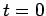the state of the system is represented by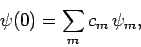(1019)

where the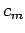are complex numbers. Thus, the initial state is some linear superposition of the unperturbed energy eigenstates. In the absence of the time-dependent perturbation, the time evolution of the system is simply (see Sect. 4.12)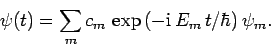(1020)

Now, the probability of finding the system in stateat timeis(1021)

since the unperturbed eigenstates are assummed to be orthonormal: i.e.,(1022)

Clearly, with, the probability of finding the system in state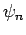at timeis exactly the same as the probability of finding the system in this state at the initial time,. However, with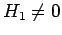, we expect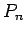--and, hence,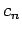--to vary with time. Thus, we can write(1023)

where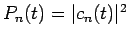. Here, we have carefully separated the fast phase oscillation of the eigenstates, which depends on the unperturbed Hamiltonian, from the slow variation of the amplitudes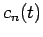, which depends entirely on the perturbation (i.e.,is constant in time if). Note that in Eq. (1023) the eigenstatesare time-independent (they are actually the eigenstates ofevaluated at the initial time,).

The time-dependent Schrödinger equation [see Eq. (199)] yields(1024)

Now, it follows from Eq. (1023) that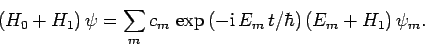(1025)

We also have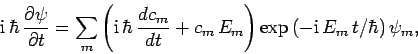(1026)

since theare time-independent. According to Eq. (1024), we can equate the right-hand sides of the previous two equations to obtain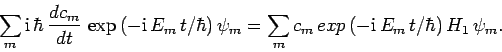(1027)

Projecting out the component of the above equation which is proportional to, using Eq. (1022), we obtain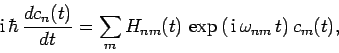(1028)

where(1029)

and(1030)

Suppose that there arelinearly independent eigenstates of the unperturbed Hamiltonian. According to Eqs. (1028), the time-dependence of the set ofcoefficients, which specify the probabilities of finding the system in these eigenstates at time, is determined bycoupled first-order differential equations. Note that Eqs. (1028) are exact--we have made no approximations at this stage. Unfortunately, we cannot generally find exact solutions to these equations. Instead, we have to obtain approximate solutions via suitable expansions in small quantities. However, for the particuilarly simple case of a two-state system (i.e.,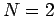), it is actually possible to solve Eqs. (1028) without approximation. This solution is of great practical importance.Next: Two-State System Up: Time-Dependent Perturbation Theory Previous: Introduction
Richard Fitzpatrick 2010-07-20